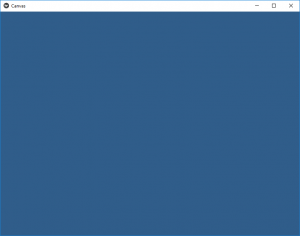# Python | Canvas in kivy

Kivy is a platform independent GUI tool in Python. As it can be run on Android, IOS, linux and Windows etc. It is basically used to develop the Android application, but it does not mean that it can not be used on Desktops applications.

Canvas:
The Canvas is the root object used for drawing by a Widget . A kivy canvas is not the place where you paint. The major problems I had at the beginning with the canvas were due to its name. Particularly considering all the buzz about HTML5 canvas. I initially think that the canvas is the paint But canvas is basically a container of instructions.
To use Canvas you must have to import:

`from kivy.graphics import Rectangle, Color`

Note: Each Widget in Kivy already has a Canvas by default. When you create a widget, you can create all the instructions needed for drawing. If self is your current widget. The instructions Color and Rectangle are automatically added to the canvas object and will be used when the window is drawn.

```Basic Approach
-> import kivy
-> import kivy App
-> import widget
-> import Canvas i.e.:
from kivy.graphics import Rectangle, Color
-> set minimum version(optional)
-> Extend the Widget class
-> Create the App Class
-> return a Widget
-> Run an instance of the class```

Implementation of the Approach –

## Python3

 `# import kivy module ` `import` `kivy ` `  `  `# this restrict the kivy version i.e ` `# below this kivy version you cannot ` `# use the app or software ` `kivy.require(``"1.9.1"``) ` `  `  `# base Class of your App inherits from the App class. ` `# app:always refers to the instance of your application ` `from` `kivy.app ``import` `App `   `# A Widget is the base building block` `# of GUI interfaces in Kivy.` `# It provides a Canvas that` `# can be used to draw on screen.` `from` `kivy.uix.widget ``import` `Widget`   `# From graphics module we are importing` `# Rectangle and Color as they are` `# basic building of canvas.` `from` `kivy.graphics ``import` `Rectangle, Color`   `# class in which we are creating the canvas` `class` `CanvasWidget(Widget):` `    `  `    ``def` `__init__(``self``, ``*``*``kwargs):`   `        ``super``(CanvasWidget, ``self``).__init__(``*``*``kwargs)`   `        ``# Arranging Canvas` `        ``with ``self``.canvas:`   `            ``Color(.``234``, .``456``, .``678``, .``8``)  ``# set the colour `   `            ``# Setting the size and position of canvas` `            ``self``.rect ``=` `Rectangle(pos ``=` `self``.center,` `                                  ``size ``=``(``self``.width ``/` `2.``,` `                                        ``self``.height ``/` `2.``))`   `            ``# Update the canvas as the screen size change` `            ``self``.bind(pos ``=` `self``.update_rect,` `                  ``size ``=` `self``.update_rect)`   `    ``# update function which makes the canvas adjustable.` `    ``def` `update_rect(``self``, ``*``args):` `        ``self``.rect.pos ``=` `self``.pos` `        ``self``.rect.size ``=` `self``.size`   `# Create the App Class` `class` `CanvasApp(App):` `    ``def` `build(``self``):` `        ``return` `CanvasWidget()`   `# run the App` `CanvasApp().run()`

Output:You can also use any other widget in canvas. In the below example we will show how to add image and change its color.
To change the color just change the canvas color that will change the image color.

## Python3

 `# import kivy module ` `import` `kivy ` `  `  `# this restrict the kivy version i.e ` `# below this kivy version you cannot ` `# use the app or software ` `kivy.require(``"1.9.1"``) ` `  `  `# base Class of your App inherits from the App class. ` `# app:always refers to the instance of your application ` `from` `kivy.app ``import` `App `   `# A Widget is the base building block` `# of GUI interfaces in Kivy.` `# It provides a Canvas that` `# can be used to draw on screen.` `from` `kivy.uix.widget ``import` `Widget`   `# From graphics module we are importing` `# Rectangle and Color as they are` `# basic building of canvas.` `from` `kivy.graphics ``import` `Rectangle, Color`   `# class in which we are creating the canvas` `class` `CanvasWidget(Widget):` `    `  `    ``def` `__init__(``self``, ``*``*``kwargs):`   `        ``super``(CanvasWidget, ``self``).__init__(``*``*``kwargs)`   `        ``# Arranging Canvas` `        ``with ``self``.canvas:`   `            ``Color(``1``, ``0``, ``0``, ``1``)  ``# set the colour `   `            ``# Setting the size and position of image` `            ``# image must be in same folder ` `            ``self``.rect ``=` `Rectangle(source ``=``'download.jpg'``,` `                                  ``pos ``=` `self``.pos, size ``=` `self``.size)`   `            ``# Update the canvas as the screen size change` `            ``# if not use this next 5 line the` `            ``# code will run but not cover the full screen` `            ``self``.bind(pos ``=` `self``.update_rect,` `                  ``size ``=` `self``.update_rect)`   `    ``# update function which makes the canvas adjustable.` `    ``def` `update_rect(``self``, ``*``args):` `        ``self``.rect.pos ``=` `self``.pos` `        ``self``.rect.size ``=` `self``.size`   `# Create the App Class` `class` `CanvasApp(App):` `    ``def` `build(``self``):` `        ``return` `CanvasWidget()`   `# run the App` `CanvasApp().run()`

Output:
Original image used in App is:Image in Canvas:Note:
Kivy drawing instructions are not automatically relative to the widgets’ position or size. You, therefore, you need to consider these factors when drawing. In order to make your drawing instructions relative to the widget, the instructions need either to be declared in the KvLang or bound to pos and size changes.

Whether you're preparing for your first job interview or aiming to upskill in this ever-evolving tech landscape, GeeksforGeeks Courses are your key to success. We provide top-quality content at affordable prices, all geared towards accelerating your growth in a time-bound manner. Join the millions we've already empowered, and we're here to do the same for you. Don't miss out - check it out now!

Previous
Next